# Quantized angles

The unit angle of the regular heptagon
In a regular heptagon all diagonals are drawn.
All sides and diagonals are extended in both directions.
Then arises the figure with a tangle of lines and angles: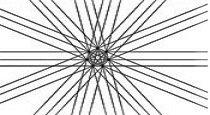This figure only contains angles 1, 2, 3, 4 or 5 × 180°/7.
I define the 180°/7 as the unit angle of the regular heptagon.
I display it as u7.

All possible trangles in the regular heptagon are of the next shapes:
(1,1,5)u7, (1,2,4)u7, (1,3,3)u7 and (2,2,3)u7.
Between () you find the three angles of the triangle
expressed in the unit angle u7.
You find the same numbers in the diagram.
The sum of the three angles in a triangle is 180° = 7 units always.
That is why in (for instance)(1,2,4)the sum of the three number is 7 again and again.
Indeed 180°/7 equals u7. So 7u7 = 180°.
The next diagram is a part of the previous figure.
In every angle you see the number of units u7: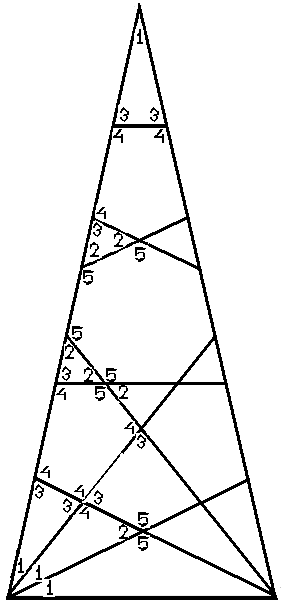In nature and daily life regular heptagons are very rare.
Look at the 20 pence coin and the 20 euro cent coin: it is a regular heptagon.
The edges has 7 notches in favor of blind.

Unit angle of any regular polygon
It is a beautiful fact, that the mentioned
quality is true for every regular polygon.
Each regular polygon has its own unit angle.
It only depends om the number of angles. Being n the unit angle equals 180°/n.

Summary
As in a regular polygon all diagonals have been drawn,
each angle is a multiple of 180°/n.
Physicits would say: the angles have been quantisized.

### I was very surprised that this property had never been discovered in the past two millennia!

The sum of the angles of a regular polygon equals (n - 2)180°.
So each angle of an empty regular polygon equals (n - 2)180°/n.

The number of diagonals is n(nn- 3)/2.

The next table shows some examples:
angles
and sides
number of
diagonals
sum of
the angles
size of the
angles in °
unit
angle
in °
related
diagram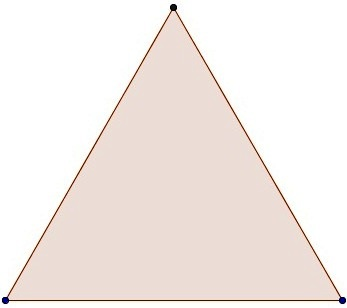equal side triangle
=
regular
triangle
30180 6060delta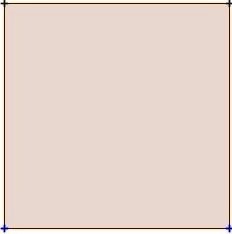squair
=
regular
423609045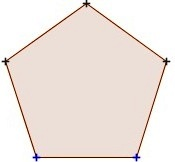regular
pentagon
=
pentagon
5554010836pentagram or
5-pointed star

only
diagonals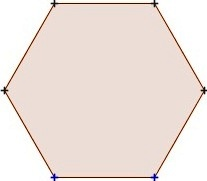regular
hexagon
6972012030Star of David

Christmas star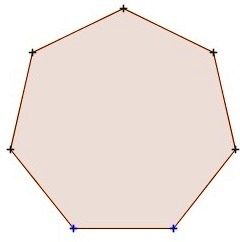regular
heptagon
714900128 + 4/725 + 5/7€ 0,20
coin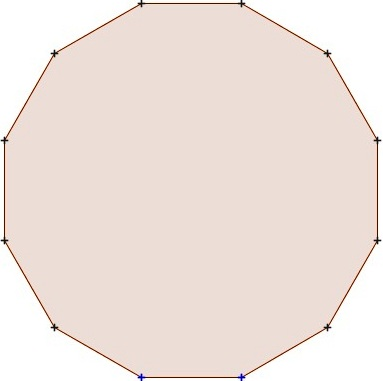regular
dodecagon
1254180015015(analog)
clock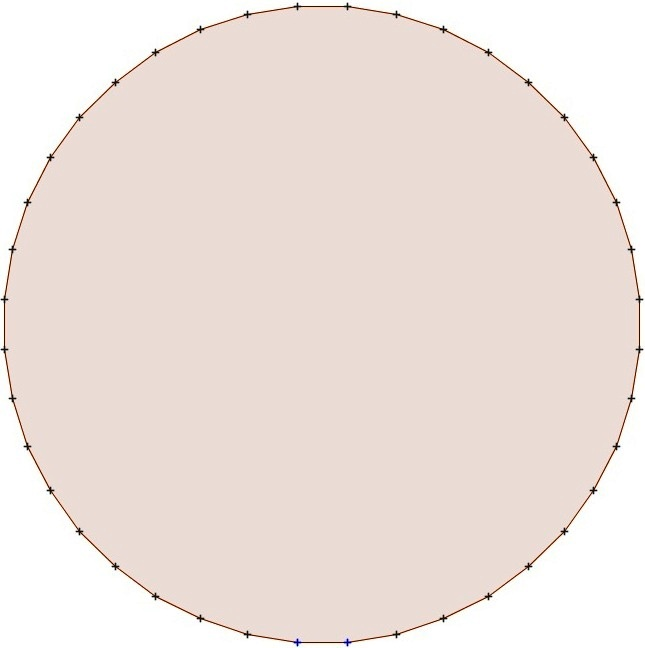regular
360-gon
36064260644401791/2almost
circle

regular
n-gon
nn(n-3)/2180(n-2)180(n-2)/n180/n

the general proof has been based on the next geometrical properties.
• 180° = n × un
• angle + supplementary angle = n × un.
• opposite angles are equal in measure.
• the measure of the central angle is exactly twice the measure of the inscribed angle.
• the inscribed angle = the unit angle
• the sum of the angles in a triangle is 180° = n × un
• the sum of the angles in a quadrangle is 360°
• the sum of the angles in an n-gon is (n - 2) un
• the regular polygon mirror symmetrical and rotational symmetrical
• that is all folks!